# High School Math : How to find an angle in a pentagon

## Example Questions

### Example Question #1 : How To Find An Angle In A Pentagon

What is the interior angle measure of any regular pentagon?

Possible Answers: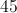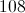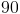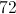Correct answer:Explanation:

To find the angle of any regular polygon you find the number of sides. For a pentagon,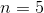.

You then subtract 2 from the number of sides yielding 3.

Take 3 and multiply it by 180 degrees to yield the total number of degrees in the regular pentagon.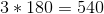Then to find one individual angle we divide 540 by the total number of angles 5,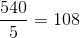The answer is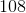.

### Example Question #2 : How To Find An Angle In A Pentagon

What is the measure of each interior angle of a regular pentagon?

Possible Answers: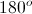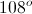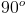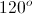Correct answer:Explanation:

The following equation can be used to determine the measure of an interior angle of a regular polygon, whereequals the number of sides.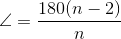In a pentagon,.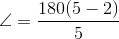Now we can solve for the angle.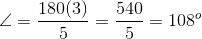### Example Question #3 : How To Find An Angle In A Pentagon

Find the interior angle of the following regular pentagon:Possible Answers: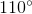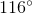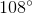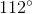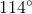Correct answer:Explanation:

The formula for the sum of the interior angles of a polygon is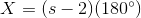,

whereis the number of sides in the polygon

Plugging in our values, we get: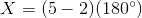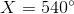Dividing the sum of the interior angles by the number of angles in the polygon, we get the value for each interior angle: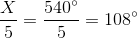### Example Question #4 : How To Find An Angle In A Pentagon

What is the measure of an interior angle of a regular pentagon?

Possible Answers:Correct answer:Explanation:

The measure of an interior angle of a regular polygon can be determined using the following equation, whereequals the number of sides: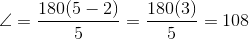### All High School Math Resources